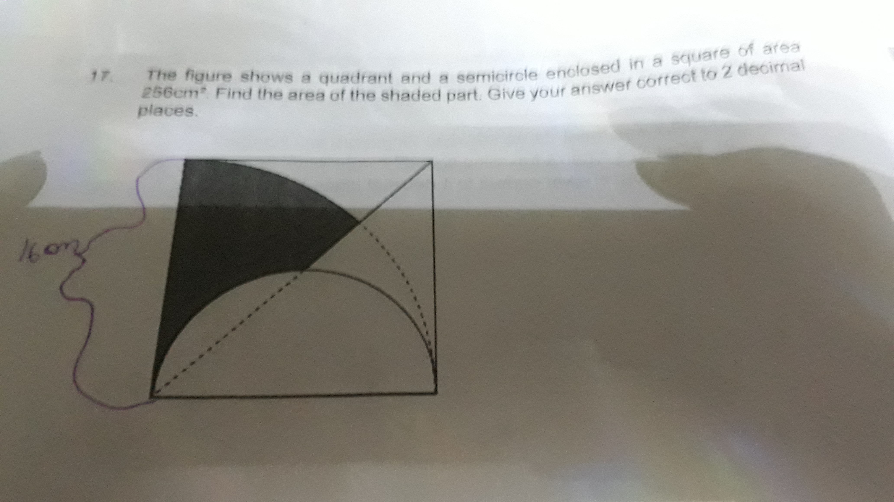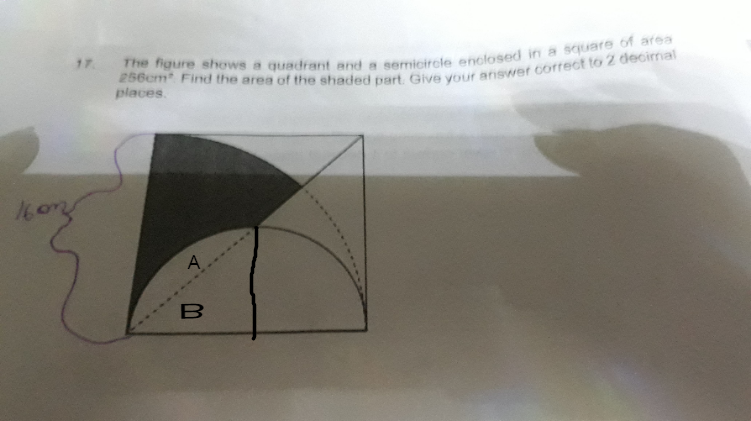# QuestionSide of square = 16cm

Area of smaller quadrant = 1/4 x 8 x 8 x pi = (16 pi )cm2

Area of triangle B = 1/2 x 8 x 8 = 32 cm2

Area of A => 16 pi cm2 – 32 cm

Area of shaded + A = 1/8 of bigger  circle

1/8 x 16 x 16 x pi = 32 pi

Shaded area + (16 pi – 32) cm2 = (32 pi) cm2

Area of Shaded area will be

(32 pi – 16 pi + 32 ) cm2

= (16 pi + 32) cm2

= 82.27 cm2

0 Replies 3 Likes ✔Accepted Answer